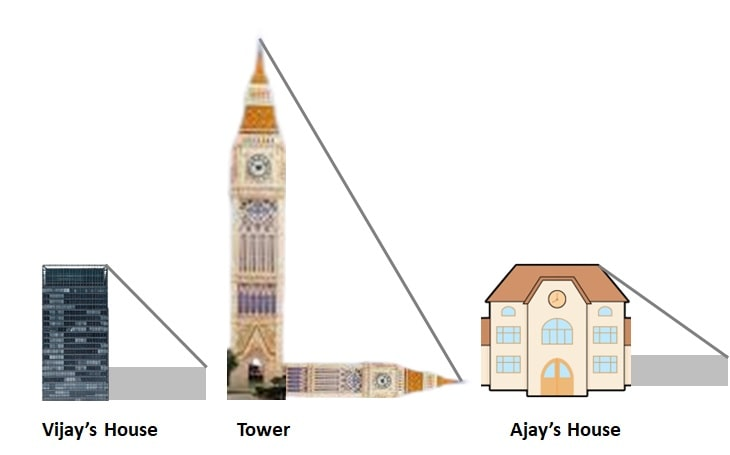Q) Vijay is trying to find the average height of a tower near his house. He is using the properties of similar triangles. The height of Vijay’s house if 20m when Vijay’s house casts a shadow 10m long on the ground. At the same time, the tower casts a shadow 50m long on the ground and the house of Ajay casts 20m shadow on the ground.1. What is the height of the tower?
a) 20m                     b) 50m                     c) 100m                     d) 200m

2. What will be the length of the shadow of the tower when Vijay’s house casts a shadow of 12m?
a) 75m                     b) 50m                     c) 45m                     d) 60m

3. What is the height of Ajay’s house?
a) 30m                     b) 40m                     c) 50m                     d) 20m

4. When the tower casts a shadow of 40m, same time what will be the length of the shadow of Ajay’s house?
a) 16m                     b) 32m                     c) 20m                     d) 8m

5. When the tower casts a shadow of 40m, same time what will be the length of the shadow of Vijay’s house?
a) 15m                     b) 32m                     c) 16m                     d) 8m

Ans: We are given following information:

Height of Vijay’s house = 20m

Shadow length of Vijay’s house = 10m

Shadow length of Tower = 50m

Shadow length of Ajay’s house = 20m

Let’s find out answers to following:

1.  Height of the tower:

By Similar triangles property:From given data, we get:∴ Height of tower = 2 x 50 = 100 m

Therefore, Option c) is correct.

2. Length of the shadow of the tower when Vijay’s house shadow is 12m:

Here, the Height of Vijay’s house is given as 20 m

and we just calculated the height of tower as 100m

By Similar triangles property:∴ Shadow length of tower == 60 m

Therefore, Option d) is correct.

3. Height of Ajay’s house?

By Similar triangles property:From given data, we get:∴ Height of Ajay’s House = 2 x 20 = 40 m

Therefore, Option b) is correct.

4. Shadow length of Ajay’s House if Tower’s shadow length is 40m:

By Similar triangles property:From given data, we get:∴ Shadow length of Ajay’s house == 16 m

Therefore, Option a) is correct.

5. Shadow length of Vijay’s House when Tower’s shadow length is 40m:

By Similar triangles property:From given data, we get:∴ Shadow length of Vijay’s house == 8 m

Therefore, Option d) is correct

Scroll to Top